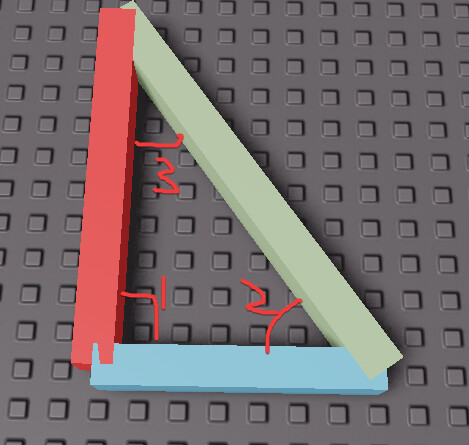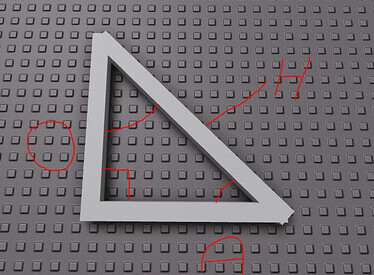# How do I find angles in a triangle?

I want to find all three angles in a triangle.

Image:

All of the sides in red I want to find using math tell me how please

I know it has something to do with:

math.sin
math.cos
math.tan

TRIED THIS AND IT DID NOT WORk

``````local Hypotenuse = workspace.Hypotenuse
local Opposite = workspace.Opposite

local MathSin = math.sin(Opposite.Position.Magnitude / Hypotenuse.Position.Magnitude)
local Angle = math.deg(math.asin(MathSin))
print(Angle)
``````

This does not represent the length of your triangle’s sides. You need to use the actual size of the parts.

Under the assumption that this is a right triangle, and that the size of any part representing the side of the triangle is given by `(1, 1, z)` where z is the length of the side, then you should be able to just do this:

(Also you should use better variable names because the definition of Adjacent and Opposite is different depending on the angle you’re looking at; but for sake of example I won’t in my example code)``````local Hypotenuse = workspace.Hypotenuse.Size.Z
local Opposite = workspace.Opposite.Size.Z

local Angle1 = 90 --// Right triangles always have a 90 degree angle
local Angle2 = math.deg(math.atan(Opposite / Adjacent))
local Angle3 = 180 - Angle1 - Angle2 --// Sum of the 3 angles in a triangle must be 180

print(Angle1, Angle2, Angle3)
``````
1 Like

But that is just always giving me 45 degrees?

In your picture, you can just use the Size of the parts. Do you not have access to the Size? Do you only have the three vertices? What?

I want it so when the player is looking at the sun it creates a blur effect and when the player is not looking at the sun it destroys it

``````while wait(.36) do
local SunDirection = game:GetService("Lighting"):GetSunDirection()
local Math = math.deg(math.atan(SunDirection.X / CurrentCameraDirection.X))
if Math > 40 and Math < 50 then
print("Looking at the sun")
end
end
``````

but the angle is effected by how much you zoom in and out

You use the length of each side which is how long each part is to figure out the angles. You also ensure that you rotated a leg relative to the other leg 90 degrees to make sure it really is a right triangle. The rest is all trigonometry lol. I recommend learning that online in practice math problems.

-Btw you can’t use those formulas because all your parts are collided towards one another, so you can’t get an accurate measurement.

If the “opposite” and “adjacent” side are the same size then it will always read 45 degrees for the two angles?

Your triangle’s legs look pretty equal to meYou shoulda started with thatIt’s much easier that this.

``````local angleBetweenTwoDirections = math.acos(cameraDirection:Dot(sunDirection))

print(“The two directions are close to lined up”)
end
``````

wait i may be worng.
but i think that would’nt work
u should be taking orientation for angles instead of position

I am looking at this but cant make much sense of it Basic Trigonometry

Stop trying to make triangles. You can calculate the angle between any two unit vectors like I showed you, using `math.acos` and `Dot`.

idk if am right.
but i think taking orientation would be a better alternative.
so its as simple as this.
(i kind of forgot how to use the lua block sorry.)

local part1 = game.Workspace.part1
local part2 = game.Workspace.part2

local angle = (part1.Orientation - part2.Orientation).X – u can use any values x or y or z.
print(angle)

– this provides the angle between two parts

Yes but I dont know when to use acos asin atan I am having trouble identifying

Like I showed youJust to repeat myself:

`local angle = math.acos(direction1.Unit:Dot(direction2.Unit))`

It will be in radians. Convert it to degrees with `local angleInDegrees = math.deg(angle)` If you really want.

don’t confuse yourself with complicated functions its just simple orientation calculation.
so if u want to find the angle between partA and partB
(forgive me but i forgot how to use codeblocks sorry!)

(just a single line of code)

local angle = (game.workspace.partA.Orientation - game.workspace.partB.Orientation).X
–x is the axis u want to calculate the angle of.

– it is actually that simple u don’t even need trignometry lol.

This only works if the orientations are aligned on the XZ plane, and if you have Parts to work with. Read OPs post earlier about what they’re actually trying to do (sun flare stuff).

Yeah it works with only parts.
But isn’t that what he asked?

Yeah it works with only parts.
But isn’t that what he asked?
Also it will work on any plane but u may get negative values.

It’s what they asked, but not what they meant. A case of the xy problem. They clarified later in the thread: